# CS B 551 ELEMENT SOF ARTIFICIAL INTELLIGENCE 1

• Slides: 83CS B 551: ELEMENT SOF ARTIFICIAL INTELLIGENCE 1 Instructor: Kris Hauser http: //cs. indiana. edu/~hauserk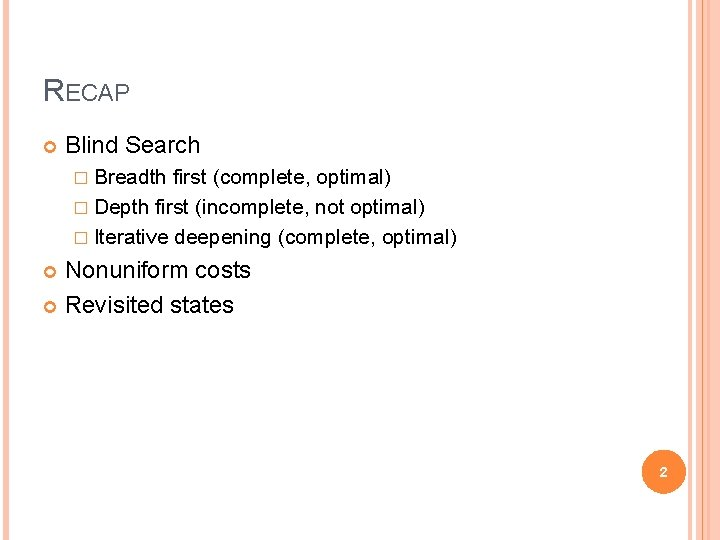RECAP Blind Search � Breadth first (complete, optimal) � Depth first (incomplete, not optimal) � Iterative deepening (complete, optimal) Nonuniform costs Revisited states 2THREE SEARCH ALGORITHMS Search #1: unit costs � With Search #2: non-unit costs, not detecting revisited states � Goal or without detecting revisited states test when node is expanded, not generated Search #3: non-unit costs, detecting revisited states � When two nodes in fringe share same state, the one with the lowest path cost is kept 3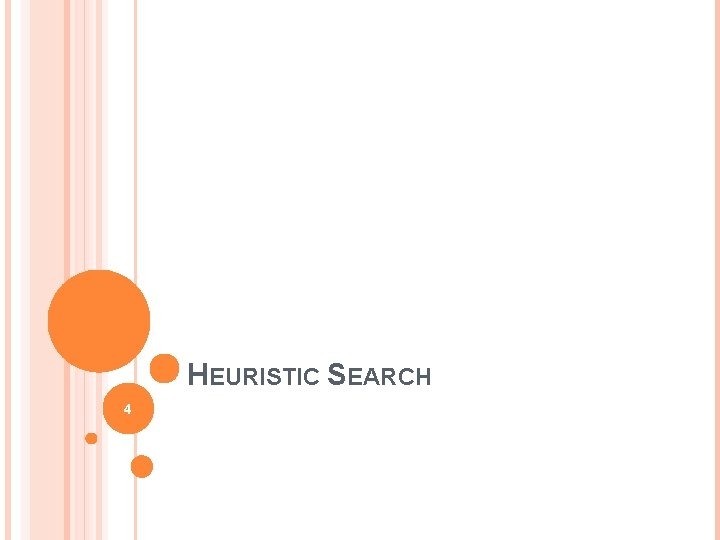HEURISTIC SEARCH 4BLIND VS. HEURISTIC STRATEGIES Blind (or un-informed) strategies do not exploit state descriptions to order FRINGE. They only exploit the positions of the nodes in the search tree Heuristic (or informed) strategies exploit state descriptions to order FRINGE (the most “promising” nodes are placed at the beginning of FRINGE) 5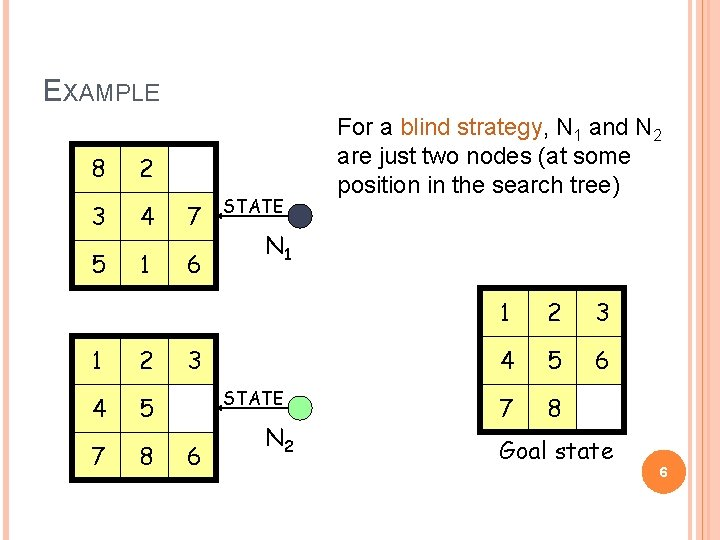EXAMPLE 8 2 3 4 7 5 1 6 1 2 4 5 7 8 STATE N 1 3 STATE 6 For a blind strategy, N 1 and N 2 are just two nodes (at some position in the search tree) N 2 1 2 3 4 5 6 7 8 Goal state 6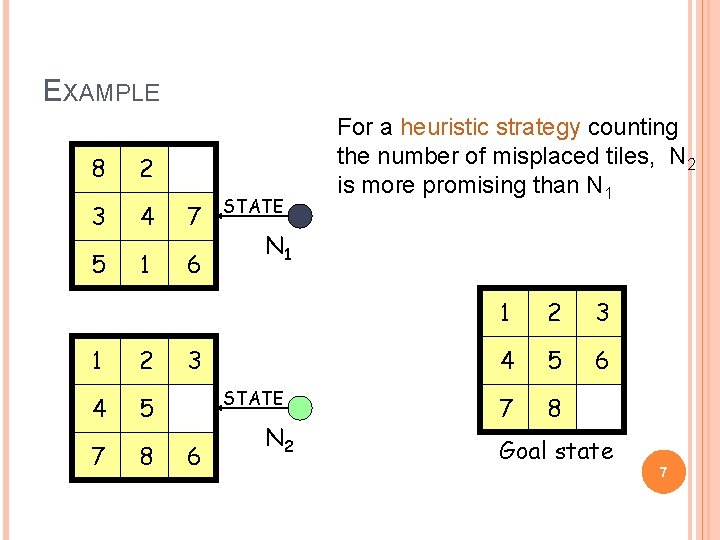EXAMPLE 8 2 3 4 7 5 1 6 1 2 4 5 7 8 STATE N 1 3 STATE 6 For a heuristic strategy counting the number of misplaced tiles, N 2 is more promising than N 1 N 2 1 2 3 4 5 6 7 8 Goal state 7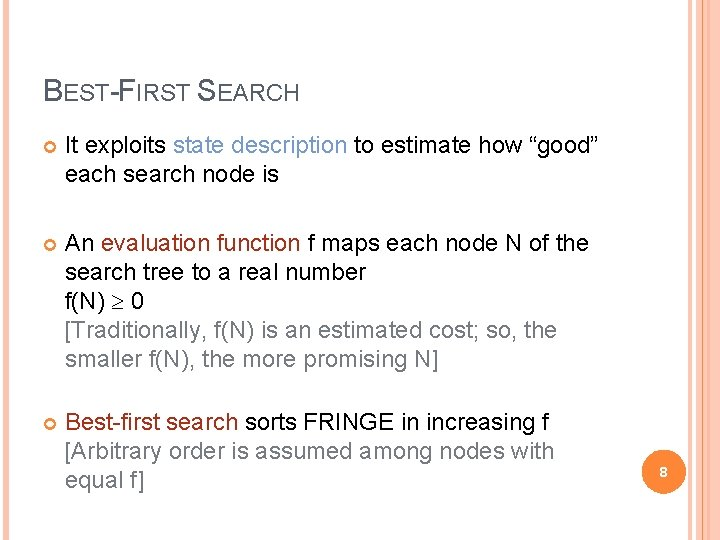BEST-FIRST SEARCH It exploits state description to estimate how “good” each search node is An evaluation function f maps each node N of the search tree to a real number f(N) 0 [Traditionally, f(N) is an estimated cost; so, the smaller f(N), the more promising N] Best-first search sorts FRINGE in increasing f [Arbitrary order is assumed among nodes with equal f] 8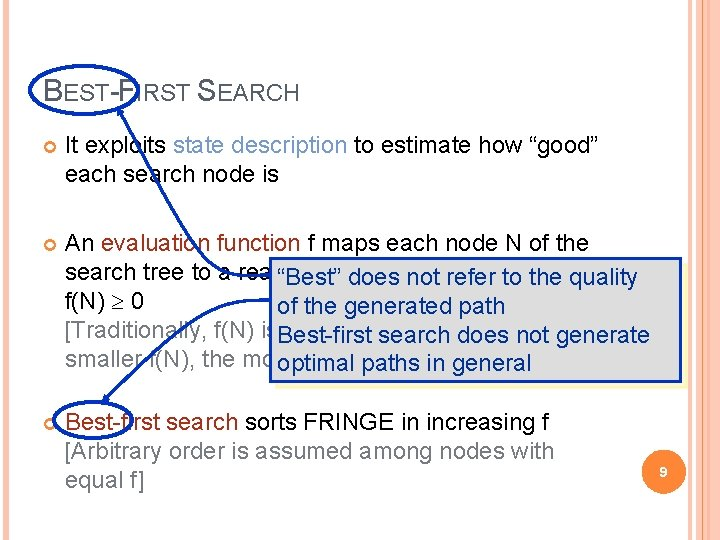BEST-FIRST SEARCH It exploits state description to estimate how “good” each search node is An evaluation function f maps each node N of the search tree to a real“Best” number does not refer to the quality f(N) 0 of the generated path [Traditionally, f(N) is. Best-first an estimated cost; so, not thegenerate search does smaller f(N), the more promising optimal paths. N]in general Best-first search sorts FRINGE in increasing f [Arbitrary order is assumed among nodes with equal f] 9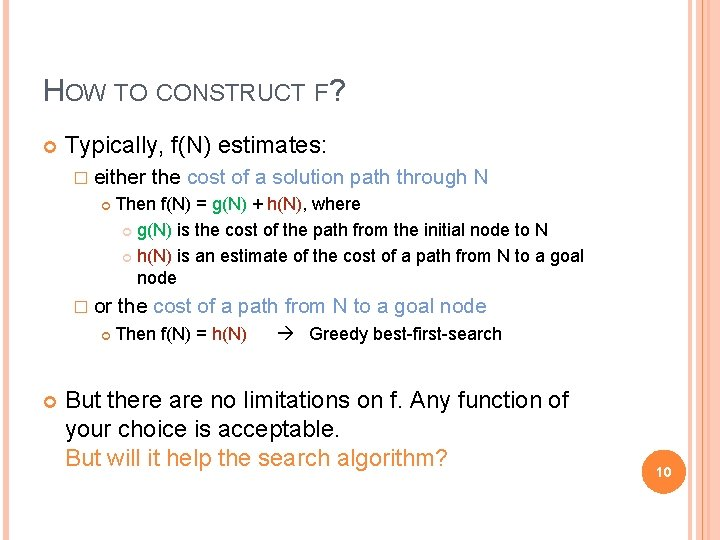HOW TO CONSTRUCT F? Typically, f(N) estimates: � either � or the cost of a solution path through N Then f(N) = g(N) + h(N), where g(N) is the cost of the path from the initial node to N h(N) is an estimate of the cost of a path from N to a goal node Then f(N) = h(N) Greedy best-first-search But there are no limitations on f. Any function of your choice is acceptable. But will it help the search algorithm? 10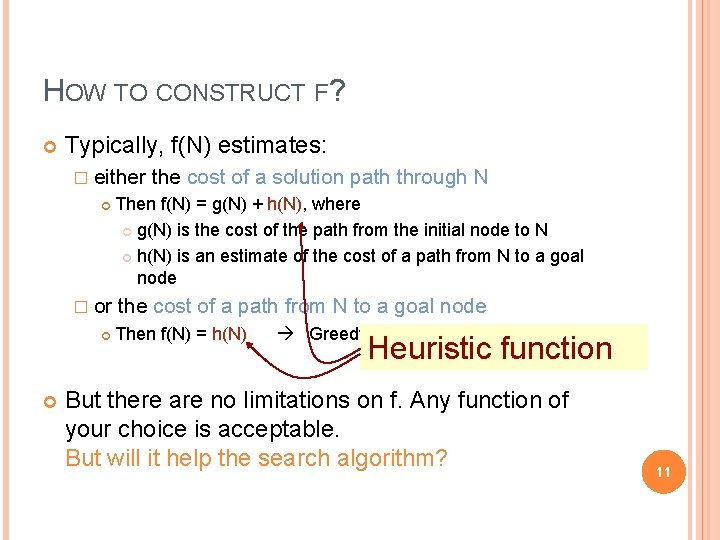HOW TO CONSTRUCT F? Typically, f(N) estimates: � either � or the cost of a solution path through N Then f(N) = g(N) + h(N), where g(N) is the cost of the path from the initial node to N h(N) is an estimate of the cost of a path from N to a goal node Then f(N) = h(N) Greedy best-first-search Heuristic function But there are no limitations on f. Any function of your choice is acceptable. But will it help the search algorithm? 11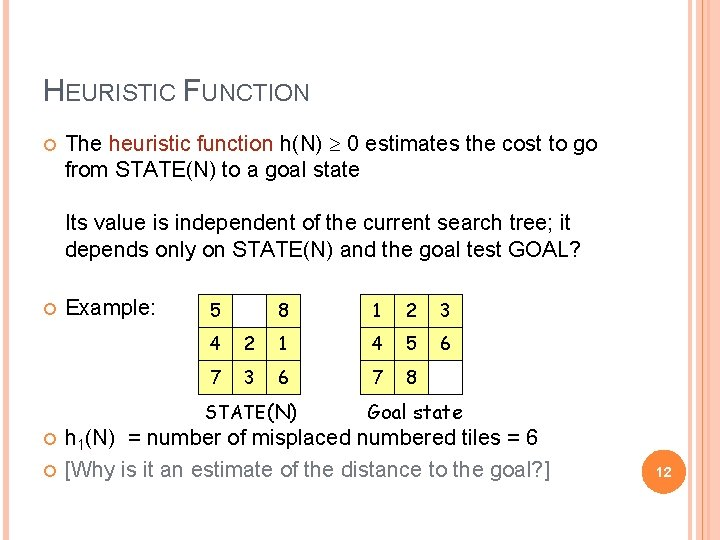HEURISTIC FUNCTION The heuristic function h(N) 0 estimates the cost to go from STATE(N) to a goal state Its value is independent of the current search tree; it depends only on STATE(N) and the goal test GOAL? Example: 5 8 1 2 3 6 4 2 1 4 5 7 3 6 7 8 STATE(N) Goal state h 1(N) = number of misplaced numbered tiles = 6 [Why is it an estimate of the distance to the goal? ] 12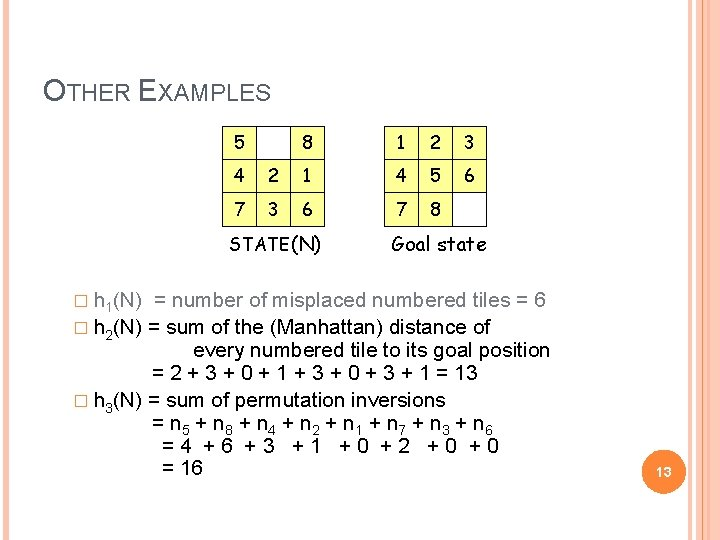OTHER EXAMPLES 5 8 1 2 3 6 4 2 1 4 5 7 3 6 7 8 STATE(N) Goal state � h 1(N) = number of misplaced numbered tiles = 6 � h 2(N) = sum of the (Manhattan) distance of every numbered tile to its goal position = 2 + 3 + 0 + 1 + 3 + 0 + 3 + 1 = 13 � h 3(N) = sum of permutation inversions = n 5 + n 8 + n 4 + n 2 + n 1 + n 7 + n 3 + n 6 =4 +6 +3 +1 +0 +2 +0 +0 = 16 138 -PUZZLE f(N) = h(N) = number of misplaced numbered tiles 3 5 3 4 2 4 2 3 3 1 0 4 5 The white tile is the empty tile 4 148 -PUZZLE f(N) = g(N) + h(N) with h(N) = number of misplaced numbered tiles 3+3 1+5 2+3 3+4 5+2 0+4 3+2 1+3 2+3 4+1 5+0 3+4 1+5 2+4 158 -PUZZLE f(N) = h(N) = S distances of numbered tiles to their goals 6 5 2 4 3 1 0 4 6 5 16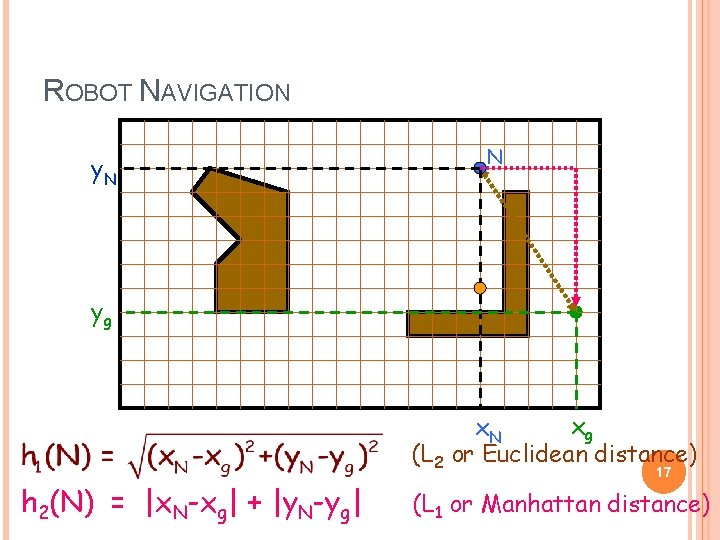ROBOT NAVIGATION y. N N yg xg x. N (L 2 or Euclidean distance) h 2(N) = |x. N-xg| + |y. N-yg| 17 (L 1 or Manhattan distance)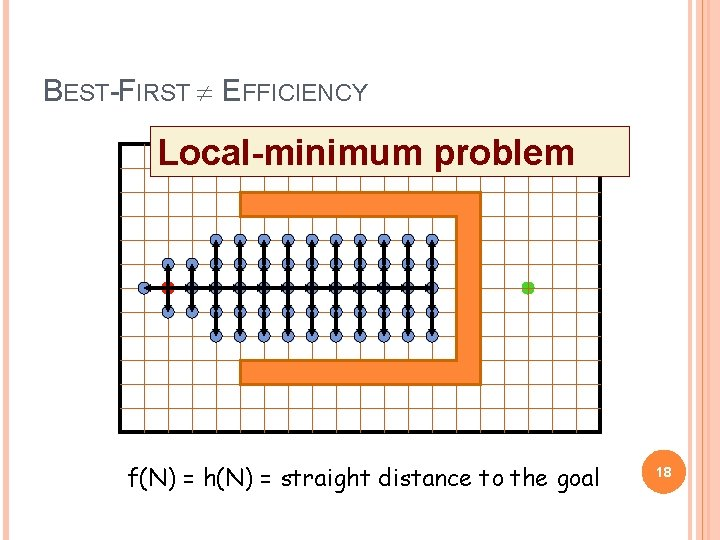BEST-FIRST EFFICIENCY Local-minimum problem f(N) = h(N) = straight distance to the goal 18ADMISSIBLE HEURISTIC Let h*(N) be the cost of the optimal path from N to a goal node The heuristic function h(N) is admissible if: 0 h(N) h*(N) An admissible heuristic function is always optimistic !ADMISSIBLE HEURISTIC Let h*(N) be the cost of the optimal path from N to a goal node The heuristic function h(N) is admissible if: 0 h(N) h*(N) An admissible heuristic function is always optimistic ! G is a goal node h(G) = 0 20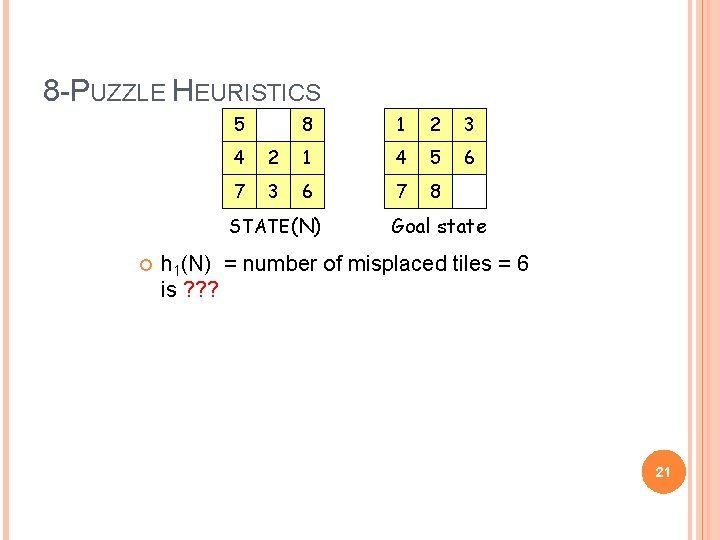8 -PUZZLE HEURISTICS 5 8 1 2 3 6 4 2 1 4 5 7 3 6 7 8 STATE(N) Goal state h 1(N) = number of misplaced tiles = 6 is ? ? ? 21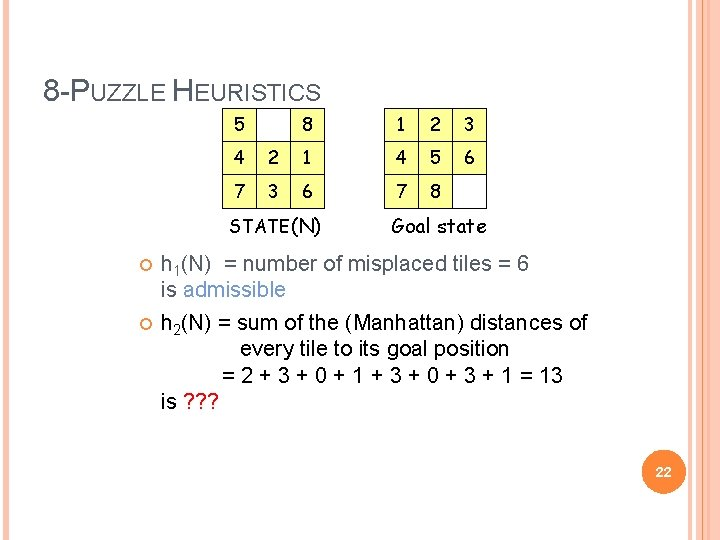8 -PUZZLE HEURISTICS 5 8 1 2 3 6 4 2 1 4 5 7 3 6 7 8 STATE(N) Goal state h 1(N) = number of misplaced tiles = 6 is admissible h 2(N) = sum of the (Manhattan) distances of every tile to its goal position = 2 + 3 + 0 + 1 + 3 + 0 + 3 + 1 = 13 is ? ? ? 22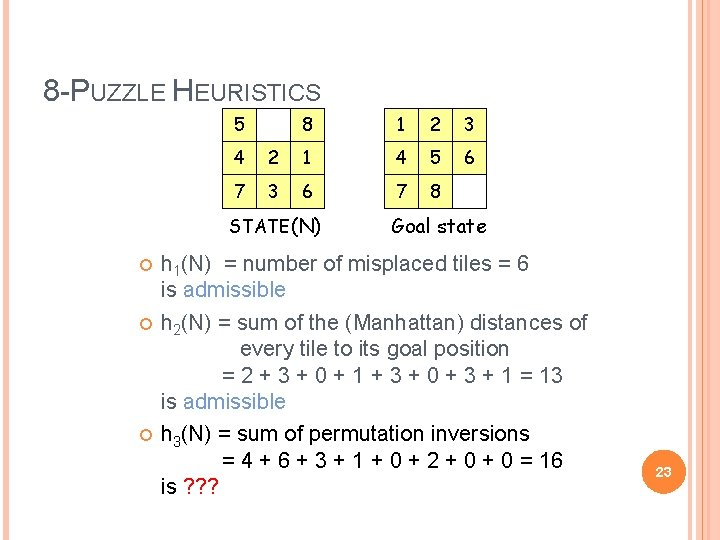8 -PUZZLE HEURISTICS 5 8 1 2 3 6 4 2 1 4 5 7 3 6 7 8 STATE(N) Goal state h 1(N) = number of misplaced tiles = 6 is admissible h 2(N) = sum of the (Manhattan) distances of every tile to its goal position = 2 + 3 + 0 + 1 + 3 + 0 + 3 + 1 = 13 is admissible h 3(N) = sum of permutation inversions = 4 + 6 + 3 + 1 + 0 + 2 + 0 = 16 is ? ? ? 23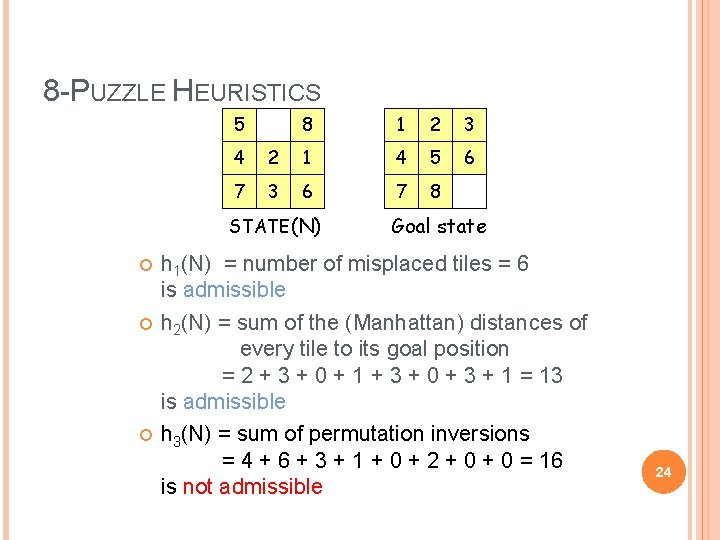8 -PUZZLE HEURISTICS 5 8 1 2 3 6 4 2 1 4 5 7 3 6 7 8 STATE(N) Goal state h 1(N) = number of misplaced tiles = 6 is admissible h 2(N) = sum of the (Manhattan) distances of every tile to its goal position = 2 + 3 + 0 + 1 + 3 + 0 + 3 + 1 = 13 is admissible h 3(N) = sum of permutation inversions = 4 + 6 + 3 + 1 + 0 + 2 + 0 = 16 is not admissible 24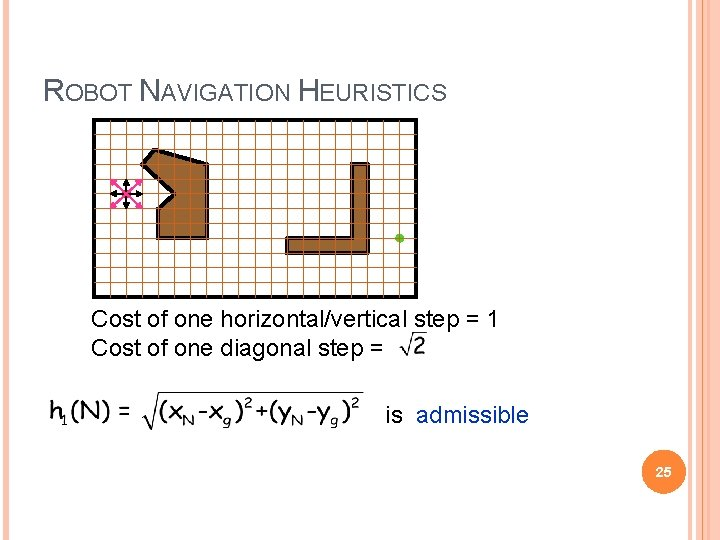ROBOT NAVIGATION HEURISTICS Cost of one horizontal/vertical step = 1 Cost of one diagonal step = is admissible 25ROBOT NAVIGATION HEURISTICS Cost of one horizontal/vertical step = 1 Cost of one diagonal step = h 2(N) = |x. N-xg| + |y. N-yg| is ? ? ? 26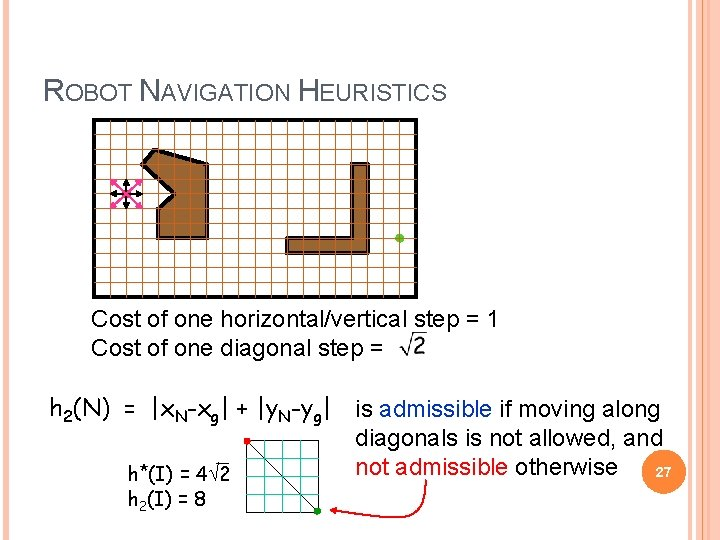ROBOT NAVIGATION HEURISTICS Cost of one horizontal/vertical step = 1 Cost of one diagonal step = h 2(N) = |x. N-xg| + |y. N-yg| is admissible if moving along diagonals is not allowed, and not admissible otherwise 27 h*(I) = 4 2 h 2(I) = 8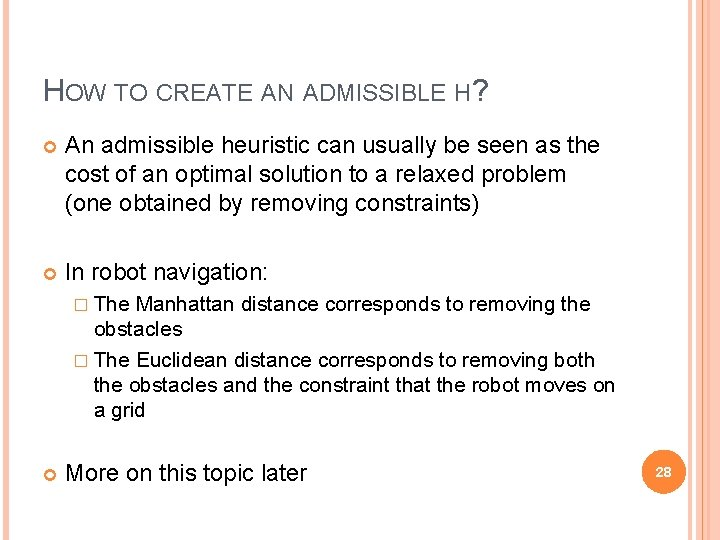HOW TO CREATE AN ADMISSIBLE H? An admissible heuristic can usually be seen as the cost of an optimal solution to a relaxed problem (one obtained by removing constraints) In robot navigation: � The Manhattan distance corresponds to removing the obstacles � The Euclidean distance corresponds to removing both the obstacles and the constraint that the robot moves on a grid More on this topic later 28A* SEARCH (MOST POPULAR ALGORITHM AI) IN f(N) = g(N) + h(N), where: g(N) = cost of best path found so far to N h(N) = admissible heuristic function for all arcs: c(N, N’) > 0 SEARCH#2 algorithm is used Best-first search is then called A* search 29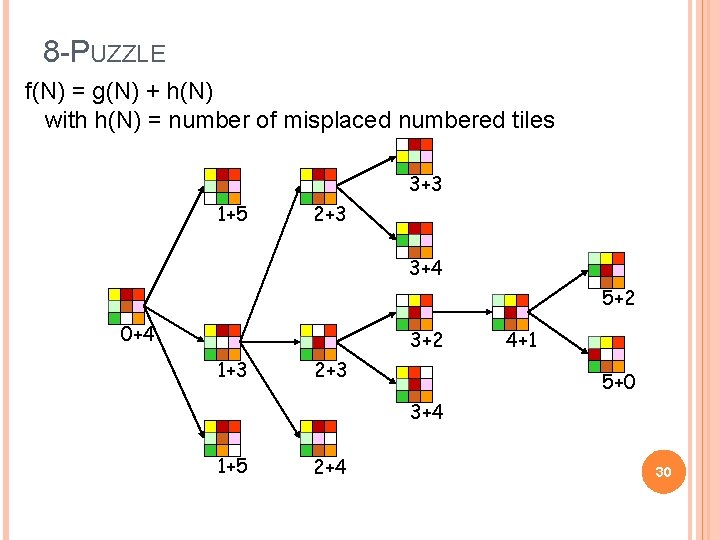8 -PUZZLE f(N) = g(N) + h(N) with h(N) = number of misplaced numbered tiles 3+3 1+5 2+3 3+4 5+2 0+4 3+2 1+3 2+3 4+1 5+0 3+4 1+5 2+4 30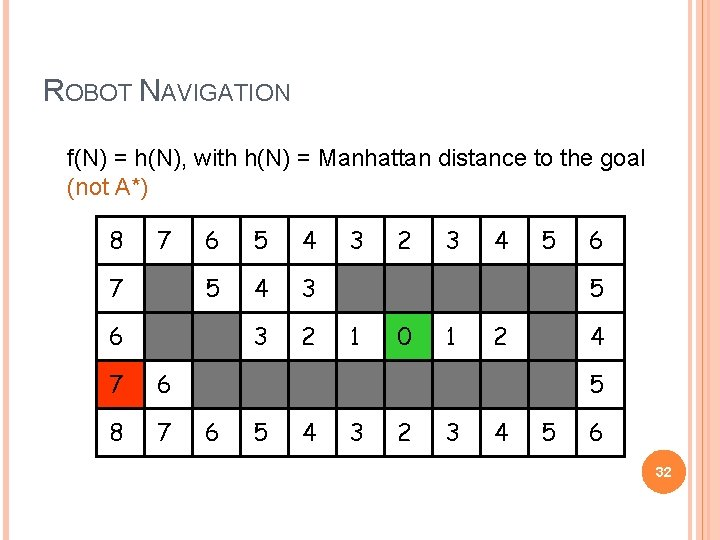ROBOT NAVIGATION f(N) = h(N), with h(N) = Manhattan distance to the goal (not A*) 8 7 7 6 5 4 3 3 2 6 7 6 8 7 3 2 3 4 5 6 5 1 0 1 2 4 5 6 5 4 3 2 3 4 5 6 32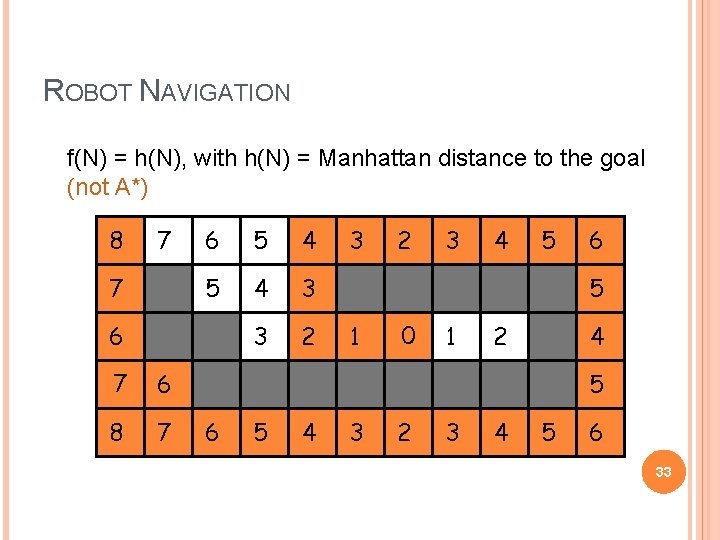ROBOT NAVIGATION f(N) = h(N), with h(N) = Manhattan distance to the goal (not A*) 8 7 7 6 5 4 3 3 2 6 77 6 8 7 3 2 3 4 5 6 5 1 00 1 2 4 5 6 5 4 3 2 3 4 5 6 33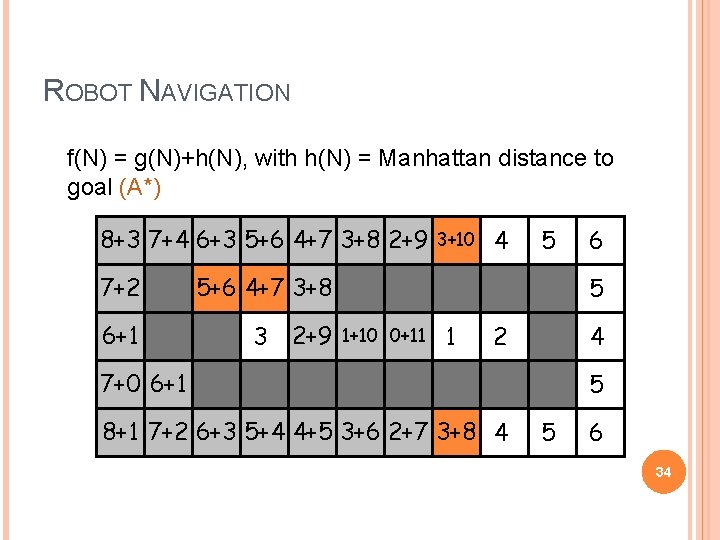ROBOT NAVIGATION f(N) = g(N)+h(N), with h(N) = Manhattan distance to goal (A*) 8+3 6+5 8 7+4 7 6+3 6 5+6 5 4+7 4 3+8 3 2+9 2 3+10 3 4 7+2 7 6+1 6 5 5+6 5 4+7 4 3+8 3 3 2+9 2 1+10 1 0+11 0 1 6 5 2 4 7+0 7 6+1 6 8+1 8 7+2 7 6+3 6 5+4 5 4+5 4 3+6 3 2+7 2 3+8 3 4 5 5 6 34RESULT #1 A* is complete and optimal [This result holds if nodes revisiting states are not discarded] 35PROOF (1/2) If a solution exists, A* terminates and returns a solution - For each node N on the fringe, f(N) = g(N)+h(N) g(N) d(N) ϵ, where d(N) is the depth of N in the tree 36PROOF (1/2) If a solution exists, A* terminates and returns a solution - For each node N on the fringe, f(N) = g(N)+h(N) g(N) d(N) ϵ, where d(N) is the depth of N in the tree K - As long as A* hasn’t terminated, a node K on the fringe lies on a solution path 37PROOF (1/2) If a solution exists, A* terminates and returns a solution - For each node N on the fringe, f(N) = g(N)+h(N) g(N) d(N) ϵ, where d(N) is the depth of N in the tree K - As long as A* hasn’t terminated, a node K on the fringe lies on a solution path - Since each node expansion increases the length of one path, K will eventually be selected for expansion, unless a solution 38 is found along another pathPROOF (2/2) Whenever A* chooses to expand a goal node, the path to this node is optimal - C*= cost of the optimal solution path - G’: non-optimal goal node in the fringe f(G’) = g(G’) + h(G’) = g(G’) C* G’ K - A node K in the fringe lies on an optimal path: f(K) = g(K) + h(K) C* - So, G’ will not be selected for expansion 39COMPLEXITY OF A* A* expands all nodes with f(N) < C* A* may expand non-solution nodes with f(N) = C* May be an exponential number of nodes unless the heuristic is sufficiently accurate � Within O(log h*(N)) of h*(N) When a problem has no solution, A* runs forever if the state space is infinite or if states can be revisited an arbitrary number of times. In other cases, it may take a huge amount of time to terminate 40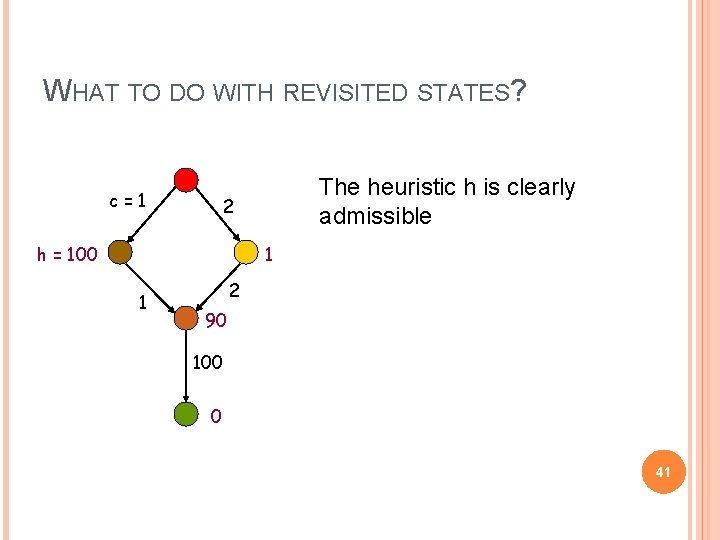WHAT TO DO WITH REVISITED STATES? c=1 The heuristic h is clearly admissible 2 h = 100 1 1 2 90 100 0 41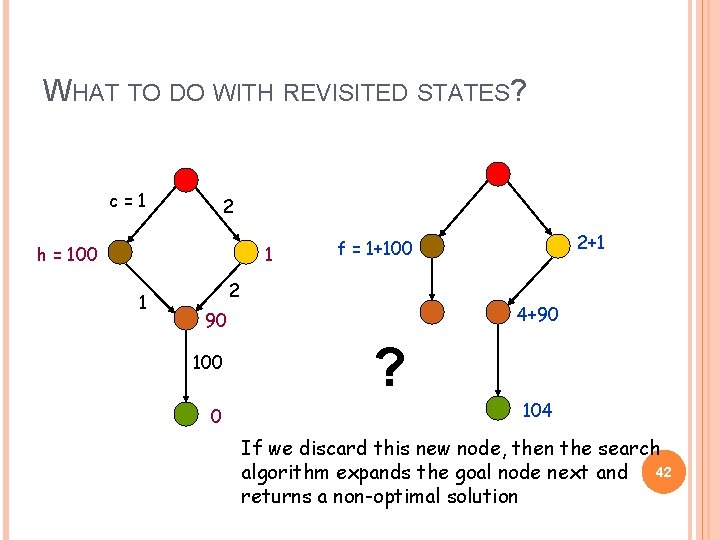WHAT TO DO WITH REVISITED STATES? c=1 2 h = 100 1 1 2 4+90 90 100 0 2+1 f = 1+100 ? 104 If we discard this new node, then the search algorithm expands the goal node next and 42 returns a non-optimal solution§ It is not harmful to discard a node revisiting a state if the cost of the new path to this state is cost of the previous path [so, in particular, one can discard a node if it re-visits a state already visited by one of its ancestors] § A* remains optimal, but states can still be revisited multiple times [the size of the search tree can still be exponential in the number of visited states] § Fortunately, for a large family of admissible heuristics – consistent heuristics – there is a much more efficient way to handle revisited states 43CONSISTENT HEURISTIC An admissible heuristic h is consistent (or monotone) if for each node N and each child N’ of N: N h(N) c(N, N’) + h(N’) c(N, N’) N’ h(N) h(N’) (triangle inequality) Intuition: a consistent heuristics becomes more 44 precise as we get deeper in the search treeCONSISTENCY VIOLATION If h tells that N is 100 units from the goal, then moving from N along an arc costing 10 units should not lead to a node N’ that h estimates to be 10 units away from the goal N c(N, N’) =10 N’ h(N’) =10 h(N) =100 (triangle inequality) 45CONSISTENT HEURISTIC (ALTERNATIVE DEFINITION) A heuristic h is consistent (or monotone) if 1. for each node N and each child N’ of N: h(N) c(N, N’) + h(N’) N 2. for each goal node G: c(N, N’) h(G) = 0 N’ h(N) h(N’) A consistent heuristic is also admissible (triangle inequality) 46ADMISSIBILITY AND CONSISTENCY A consistent heuristic is also admissible An admissible heuristic may not be consistent, but many admissible heuristics are consistent 478 -PUZZLE 5 N 1 2 3 6 4 2 1 4 5 7 3 6 7 8 STATE(N) c(N, N’) N’ 8 goal h(N) h(N’) h(N) c(N, N’) + h(N’) § h 1(N) = number of misplaced tiles § h 2(N) = sum of the (Manhattan) distances of every tile to its goal position are both consistent (why? ) 48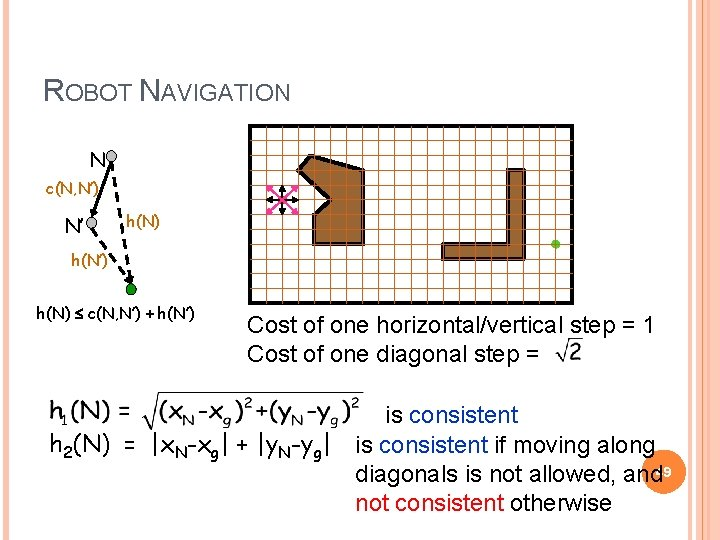ROBOT NAVIGATION N c(N, N’) N’ h(N) h(N’) h(N) c(N, N’) + h(N’) Cost of one horizontal/vertical step = 1 Cost of one diagonal step = is consistent h 2(N) = |x. N-xg| + |y. N-yg| is consistent if moving along diagonals is not allowed, and 49 not consistent otherwise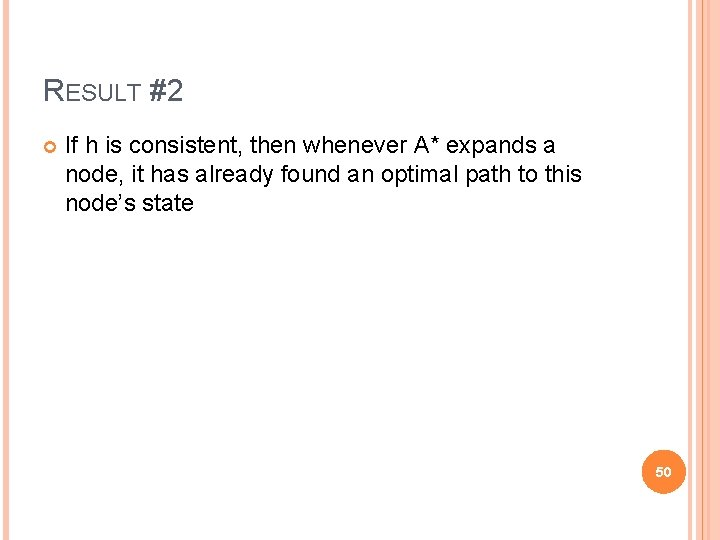RESULT #2 If h is consistent, then whenever A* expands a node, it has already found an optimal path to this node’s state 50N PROOF (1/2) 1. N’ Consider a node N and its child N’ Since h is consistent: h(N) c(N, N’)+h(N’) f(N) = g(N)+h(N) g(N)+c(N, N’)+h(N’) = f(N’) So, f is non-decreasing along any path 51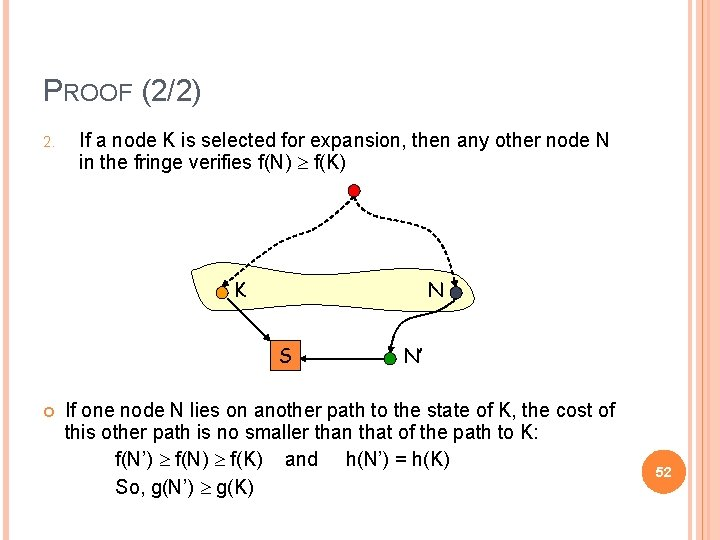PROOF (2/2) 2. If a node K is selected for expansion, then any other node N in the fringe verifies f(N) f(K) K N S N’ If one node N lies on another path to the state of K, the cost of this other path is no smaller than that of the path to K: f(N’) f(N) f(K) and h(N’) = h(K) So, g(N’) g(K) 52Result #2 PROOF (2/2) If h is consistent, then whenever A* expands a node, has Kalready found an optimal path thisnode’s 2. If aitnode is selected for expansion, then anyto other N in state the fringe verifies f(N) f(K) K N S N’ If one node N lies on another path to the state of K, the cost of this other path is no smaller than that of the path to K: f(N’) f(N) f(K) and h(N’) = h(K) So, g(N’) g(K) 53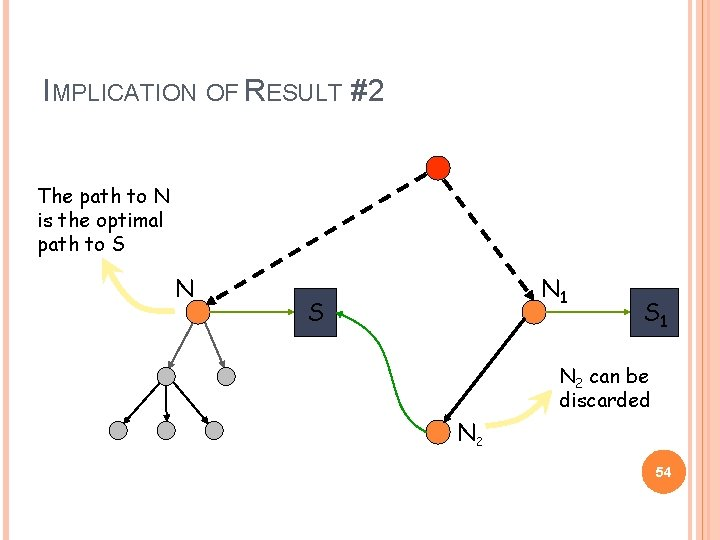IMPLICATION OF RESULT #2 The path to N is the optimal path to S N N 1 S S 1 N 2 can be discarded N 2 54REVISITED STATES WITH CONSISTENT HEURISTIC (SEARCH#3) When a node is expanded, store its state into CLOSED When a new node N is generated: � If STATE(N) is in CLOSED, discard N � If there exists a node N’ in the fringe such that STATE(N’) = STATE(N), discard the node – N or N’ – with the largest f (or, equivalently, g) 55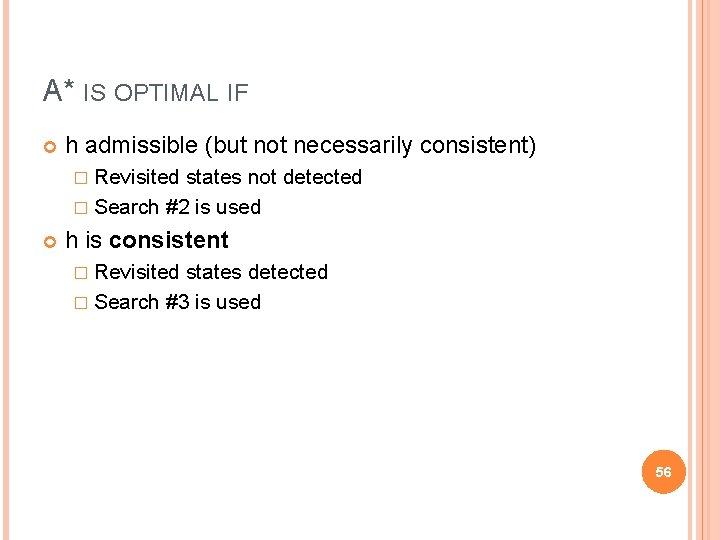A* IS OPTIMAL IF h admissible (but not necessarily consistent) � Revisited states not detected � Search #2 is used h is consistent � Revisited states detected � Search #3 is used 56HEURISTIC ACCURACY Let h 1 and h 2 be two consistent heuristics such that for all nodes N: h 1(N) h 2 is said to be more accurate (or more informed) than h 1 5 8 1 2 3 6 4 2 1 4 5 7 3 6 7 8 STATE(N) § § Goal state § h 1(N) = number of misplaced tiles h 2(N) = sum of distances of every tile to its goal position h 2 is more accurate than h 1 57RESULT #3 Let h 2 be more accurate than h 1 Let A 1* be A* using h 1 and A 2* be A* using h 2 Whenever a solution exists, all the nodes expanded by A 2*, except possibly for some nodes such that f 1(N) = f 2(N) = C* (cost of optimal solution) are also expanded by A 1* 58PROOF C* = h*(initial-node) [cost of optimal solution] Every node N such that f(N) C* is eventually expanded. No node N such that f(N) > C* is ever expanded Every node N such that h(N) C* g(N) is eventually expanded. So, every node N such that h 2(N) C* g(N) is expanded by A 2*. Since h 1(N) h 2(N), N is also expanded by A 1* If there are several nodes N such that f 1(N) = f 2(N) = C* (such nodes include the optimal goal nodes, if there exists a solution), A 1* and A 2* may or may not expand them in the same order (until one goal node is expanded) 59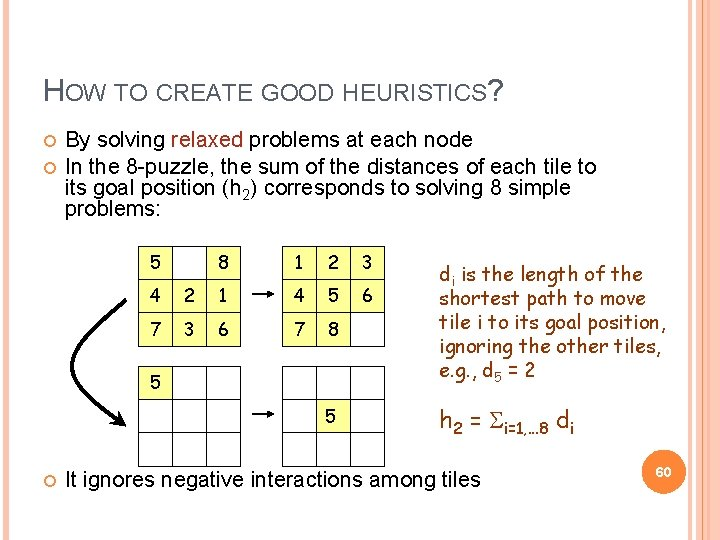HOW TO CREATE GOOD HEURISTICS? By solving relaxed problems at each node In the 8 -puzzle, the sum of the distances of each tile to its goal position (h 2) corresponds to solving 8 simple problems: 5 8 1 2 3 6 4 2 1 4 5 7 3 6 7 8 di is the length of the shortest path to move tile i to its goal position, ignoring the other tiles, e. g. , d 5 = 2 5 h 2 = Si=1, . . . 8 di 5 It ignores negative interactions among tiles 60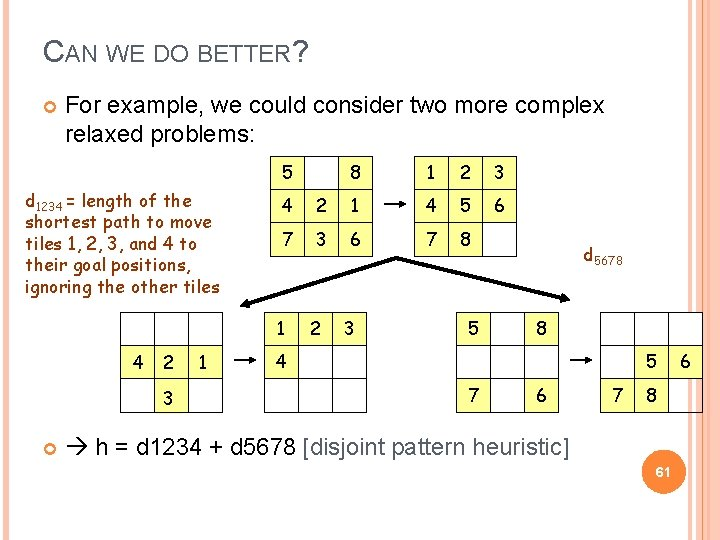CAN WE DO BETTER? For example, we could consider two more complex relaxed problems: 5 d 1234 = length of the shortest path to move tiles 1, 2, 3, and 4 to their goal positions, ignoring the other tiles 2 3 1 1 2 3 6 4 2 1 4 5 7 3 6 7 8 1 4 8 2 3 5 d 5678 8 4 5 7 6 7 8 h = d 1234 + d 5678 [disjoint pattern heuristic] 61 6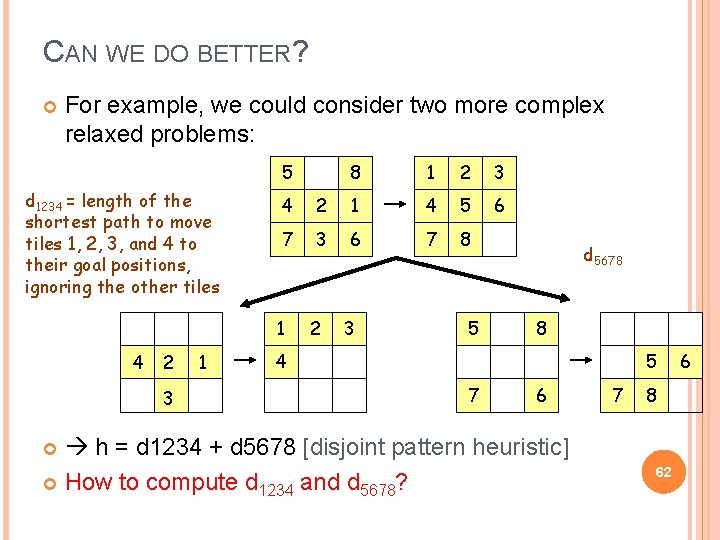CAN WE DO BETTER? For example, we could consider two more complex relaxed problems: 5 d 1234 = length of the shortest path to move tiles 1, 2, 3, and 4 to their goal positions, ignoring the other tiles 2 3 1 1 2 3 6 4 2 1 4 5 7 3 6 7 8 1 4 8 2 3 5 d 5678 8 4 5 7 6 h = d 1234 + d 5678 [disjoint pattern heuristic] How to compute d 1234 and d 5678? 7 8 62 6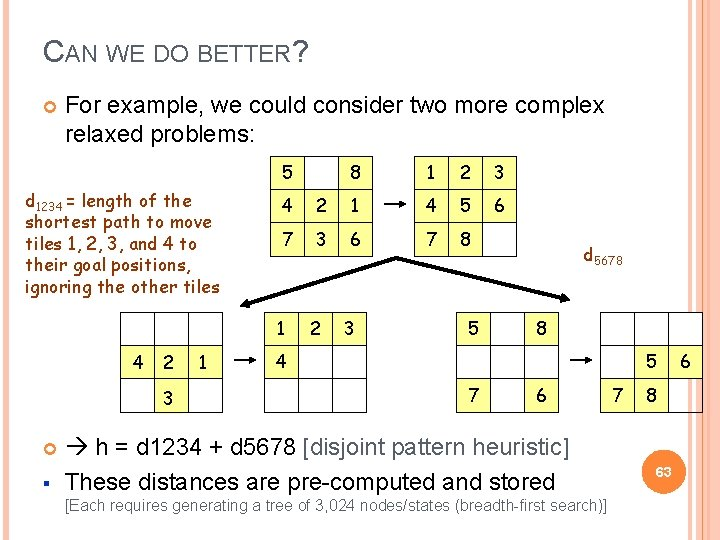CAN WE DO BETTER? For example, we could consider two more complex relaxed problems: 5 d 1234 = length of the shortest path to move tiles 1, 2, 3, and 4 to their goal positions, ignoring the other tiles 2 3 § 1 1 2 3 6 4 2 1 4 5 7 3 6 7 8 1 4 8 2 3 5 d 5678 8 4 5 7 6 h = d 1234 + d 5678 [disjoint pattern heuristic] These distances are pre-computed and stored [Each requires generating a tree of 3, 024 nodes/states (breadth-first search)] 7 8 63 6CAN WE DO BETTER? For example, we could consider two more complex relaxed problems: 5 d 1234 = length of the shortest path to move tiles 1, 2, 3, and 4 to their goal positions, ignoring the other tiles 4 2 8 1 2 3 1 4 5 6 Several order-of-magnitude speedups 7 3 6 7 8 d for the 15 - and 24 -puzzle (see R&N) 5678 1 4 2 3 § 1 2 3 5 8 4 5 7 6 h = d 1234 + d 5678 [disjoint pattern heuristic] These distances are pre-computed and stored [Each requires generating a tree of 3, 024 nodes/states (breadth-first search)] 7 8 64 6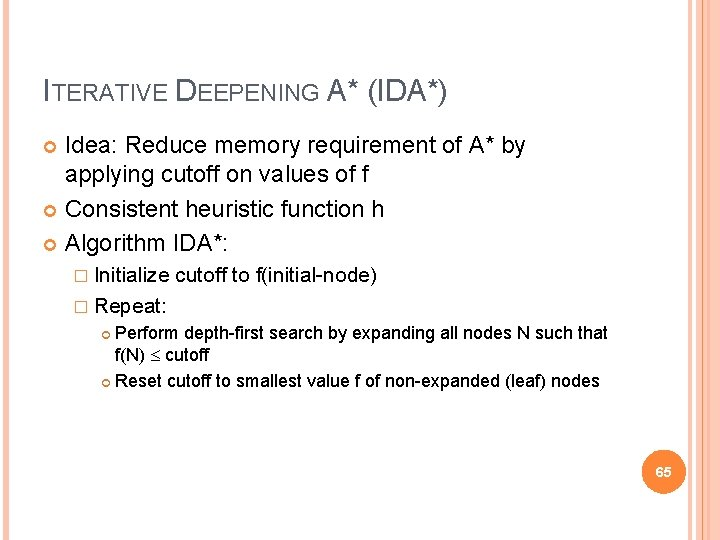ITERATIVE DEEPENING A* (IDA*) Idea: Reduce memory requirement of A* by applying cutoff on values of f Consistent heuristic function h Algorithm IDA*: � Initialize cutoff to f(initial-node) � Repeat: Perform depth-first search by expanding all nodes N such that f(N) cutoff Reset cutoff to smallest value f of non-expanded (leaf) nodes 658 -PUZZLE f(N) = g(N) + h(N) with h(N) = number of misplaced tiles 4 Cutoff=4 6 668 -PUZZLE f(N) = g(N) + h(N) with h(N) = number of misplaced tiles 4 Cutoff=4 4 6 6 678 -PUZZLE f(N) = g(N) + h(N) with h(N) = number of misplaced tiles 4 Cutoff=4 4 5 6 6 68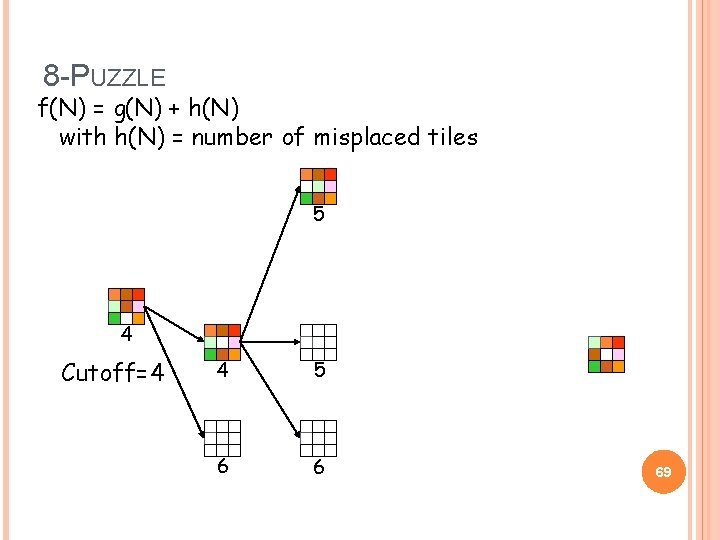8 -PUZZLE f(N) = g(N) + h(N) with h(N) = number of misplaced tiles 5 4 Cutoff=4 4 5 6 6 69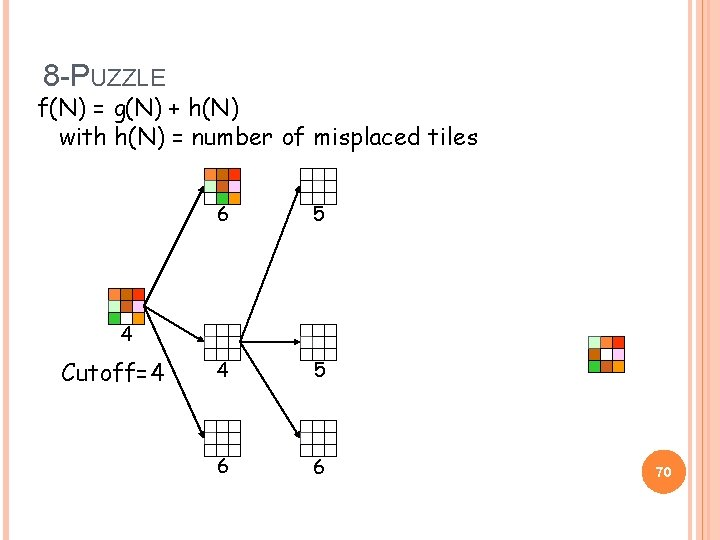8 -PUZZLE f(N) = g(N) + h(N) with h(N) = number of misplaced tiles 6 5 4 5 6 6 4 Cutoff=4 708 -PUZZLE f(N) = g(N) + h(N) with h(N) = number of misplaced tiles 4 Cutoff=5 6 71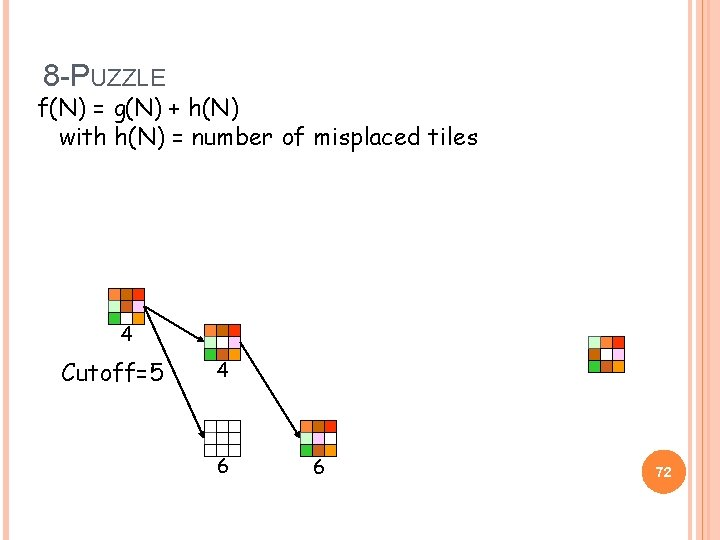8 -PUZZLE f(N) = g(N) + h(N) with h(N) = number of misplaced tiles 4 Cutoff=5 4 6 6 72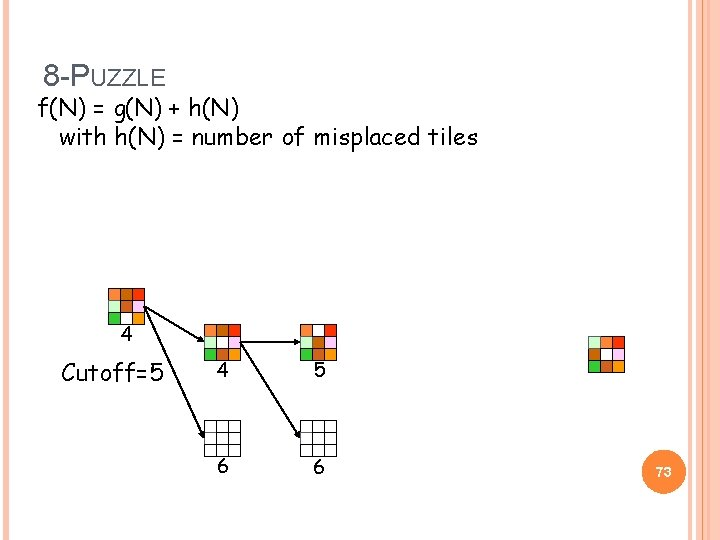8 -PUZZLE f(N) = g(N) + h(N) with h(N) = number of misplaced tiles 4 Cutoff=5 4 5 6 6 73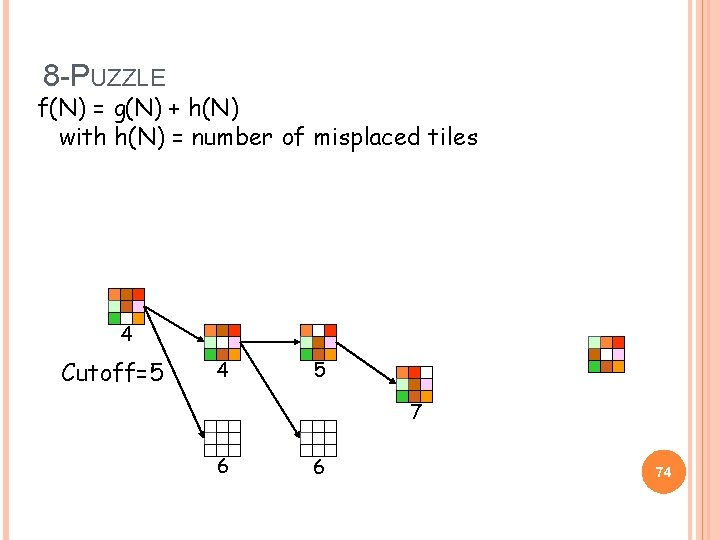8 -PUZZLE f(N) = g(N) + h(N) with h(N) = number of misplaced tiles 4 Cutoff=5 4 5 7 6 6 748 -PUZZLE f(N) = g(N) + h(N) with h(N) = number of misplaced tiles 4 Cutoff=5 5 4 5 7 6 6 758 -PUZZLE f(N) = g(N) + h(N) with h(N) = number of misplaced tiles 4 Cutoff=5 5 4 5 5 7 6 6 76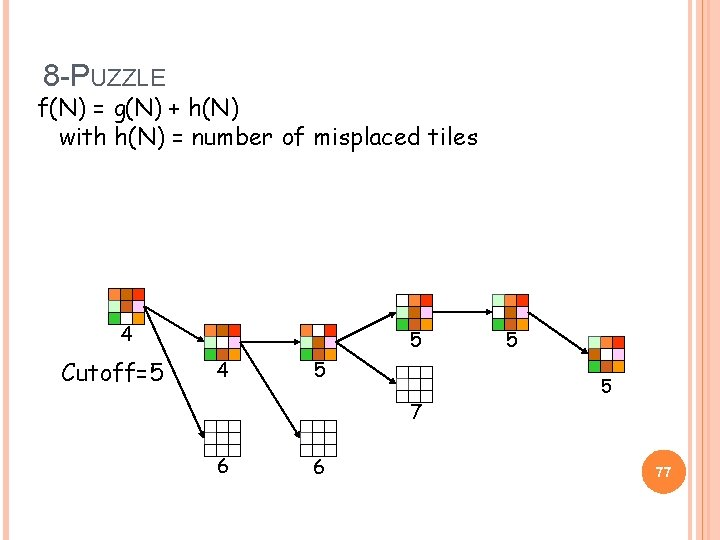8 -PUZZLE f(N) = g(N) + h(N) with h(N) = number of misplaced tiles 4 Cutoff=5 5 4 5 7 6 6 5 5 77ADVANTAGES/DRAWBACKS OF IDA* Advantages: � Still complete and optimal � Requires less memory than A* � Avoid the overhead to sort the fringe Drawbacks: � Can’t avoid revisiting states not on the current path � Available memory is poorly used � Non-unit costs? 78MEMORY-BOUNDED SEARCH Proceed like A* until memory is full � No more nodes can be added to search tree � Drop node in fringe with highest f(N) � Place parent back in fringe with “backed-up” f(P) min(f(P), f(N)) Extreme example: RBFS � Only keeps nodes in path to current node 79RECAP Proving properties of A* performance Admissible heuristics: optimality Consistent heuristics: revisited states 80NEXT CLASS Beyond classical search R&N 4. 1 -5, 6. 1 -3 81EFFECTIVE BRANCHING FACTOR It is used as a measure the effectiveness of a heuristic Let n be the total number of nodes expanded by A* for a particular problem and d the depth of the solution The effective branching factor b* is defined by n = 1 + b* + (b*)2 +. . . + (b*)d 82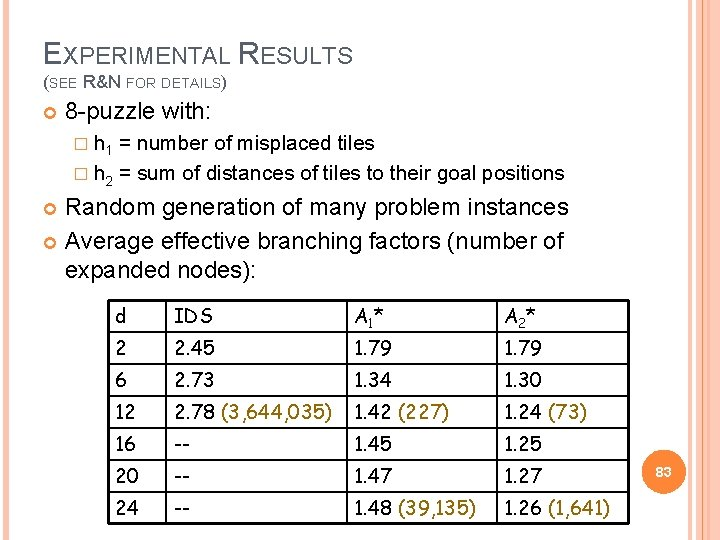EXPERIMENTAL RESULTS (SEE R&N FOR DETAILS) 8 -puzzle with: � h 1 = number of misplaced tiles � h 2 = sum of distances of tiles to their goal positions Random generation of many problem instances Average effective branching factors (number of expanded nodes): d IDS A 1 * A 2* 2 2. 45 1. 79 6 2. 73 1. 34 1. 30 12 2. 78 (3, 644, 035) 1. 42 (227) 1. 24 (73) 16 -- 1. 45 1. 25 20 -- 1. 47 1. 27 24 -- 1. 48 (39, 135) 1. 26 (1, 641) 83